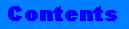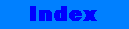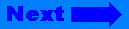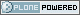# ICTP Portal

##### Sections
« December 2023 »
Su Mo Tu We Th Fr Sa
1 2
3 4 5 6 7 8 9
10 11 12 13 14 15 16
17 18 19 20 21 22 23
24 25 26 27 28 29 30
31

You are here: Home bitset

# bitset## bitset

`Container`

### Summary

A template class and related functions for storing and manipulating fixed-size sequences of bits.

### Data Type and Member Function Indexes(exclusive of constructors and destructors)

 Member Functions any() count() flip() none() operator!=() operator&() operator&=() operator<<() operator<<=() operator=() operator==() >()">operator>>() >=()">operator>>=() operator^() operator^=() operator~() reset() set() size() test() to_string() to_ulong()

### Synopsis

```#include <bitset>

template <size_t N>
class bitset ;
```

### Description

bitset<size_t N> is a class that describes objects that can store a sequence consisting of a fixed number of bits, N. Each bit represents either the value zero (reset) or one (set) and has a non-negative position pos.

### Errors and exceptions

Bitset constructors and member functions may report the following three types of errors -- each associated with a distinct exception:

• invalid-argument error or invalid_argument() exception;

• out-of-range error or out_of_range() exception;

• overflow error or over-flow_error() exception;

If exceptions are not supported on your compiler, you will get an assertion failure instead of an exception.

### Interface

```template <size_t N>
class bitset {

public:

// bit reference:

class reference {
friend class bitset<N>;
public:

~reference();
reference& operator= (bool);
reference& operator= (const reference&);
bool operator~() const;
operator bool() const;
reference& flip();
};

// Constructors
bitset ();
bitset (unsigned long);
explicit bitset (const string&, size_t = 0,
size_t = (size_t)-1);
bitset (const bitset<N>&);
bitset<N>& operator= (const bitset<N>&);

// Bitwise Operators and Bitwise Operator Assignment
bitset<N>& operator&= (const bitset<N>&);
bitset<N>& operator|= (const bitset<N>&);
bitset<N>& operator^= (const bitset<N>&);
bitset<N>& operator<<= (size_t);
bitset<N>& operator>>= (size_t);

// Set, Reset, Flip
bitset<N>& set ();
bitset<N>& set (size_t, int = 1);
bitset<N>& reset ();
bitset<N>& reset (size_t);
bitset<N> operator~() const;
bitset<N>& flip ();
bitset<N>& flip (size_t);

// element access
reference operator[] (size_t);
unsigned long to_ulong() const;
string to_string() const;
size_t count() const;
size_t size() const;
bool operator== (const bitset<N>&) const;
bool operator!= (const bitset<N>&) const;
bool test (size_t) const;
bool any() const;
bool none() const;
bitset<N> operator<< (size_t) const;
bitset<N> operator>> (size_t) const;

};

// Non-member operators
template <size_t N>
bitset<N> operator& (const bitset<N>&, const bitset<N>&);

template <size_t N>
bitset<N> operator| (const bitset<N>&, const bitset<N>&);

template <size_t N>
bitset<N> operator^ (const bitset<N>&, const bitset<N>&);

template <size_t N>
istream& operator>> (istream&, bitset<N>&);

template <size_t N>
ostream& operator<< (ostream&, const bitset<N>&);
```

### Constructors

`bitset();`

Constructs an object of class bitset<N>, initializing all bit values to zero.

`bitset(unsigned long val);`

Constructs an object of class bitset<N>, initializing the first M bit values to the corresponding bits in val. M is the smaller of N and the value CHAR_BIT * sizeof(unsigned long). If M < N, remaining bit positions are initialized to zero. Note: CHAR_BIT is defined in <climits>.

```explicit
bitset(const string& str, size_t pos = 0,
size_t n = (size_t)-1);```

Determines the effective length rlen of the initializing string as the smaller of n and str.size() - pos. The function throws an invalid_argument exception if any of the rlen characters in str, beginning at position pos,is other than 0 or 1. Otherwise, the function constructs an object of class bitset<N>, initializing the first M bit positions to values determined from the corresponding characters in the string str. M is the smaller of N and rlen. This constructor requires that pos <= str.size(), otherwise it throws an out_of_range exception.

`bitset(const bitset<N>& rhs);`

Copy constructor. Creates a copy of rhs.

### Assignment Operator

```bitset<N>&
operator=(const bitset<N>& rhs);```

Erases all bits in self, then inserts into self a copy of each bit in rhs. Returns a reference to *this.

### Operators

```bool
operator==(const bitset<N>& rhs) const;```

Returns true if the value of each bit in *this equals the value of each corresponding bit in rhs. Otherwise returns false.

```bool
operator!=(const bitset<N>& rhs) const;```

Returns true if the value of any bit in *this is not equal to the value of the corresponding bit in rhs. Otherwise returns false.

```bitset<N>&
operator&=(const bitset<N>& rhs);```

Clears each bit in *this for which the corresponding bit in rhs is clear and leaves all other bits unchanged. Returns *this.

```bitset<N>&
operator|=(const bitset<N>& rhs);```

Sets each bit in *this for which the corresponding bit in rhs is set, and leaves all other bits unchanged. Returns *this.

```bitset<N>&
operator^=(const bitset<N>& rhs);```

Toggles each bit in *this for which the corresponding bit in rhs is set, and leaves all other bits unchanged. Returns *this.

```bitset<N>&
operator<<=(size_t pos);```

Replaces each bit at position I with 0 if I < pos or with the value of the bit at I - pos if I >= pos. Returns *this.

>=()">
```bitset<N>&
operator>>=(size_t pos);```

Replaces each bit at position I with 0 if pos >= N-I or with the value of the bit at position I + pos if pos < N-I. Returns *this.

>()">
```bitset<N>&
operator>>(size_t pos) const;```

Returns bitset<N>(*this) >>= pos.

```bitset<N>&
operator<<(size_t pos) const;```

Returns bitset<N>(*this) <<= pos.

```bitset<N>
operator~() const;```

Returns the bitset that is the logical complement of each bit in *this.

```bitset<N>
operator&(const bitset<N>& lhs,
const bitset<N>& rhs);```

lhs gets logical AND of lhs with rhs.

```bitset<N>
operator|(const bitset<N>& lhs,
const bitset<N>& rhs);```

lhs gets logical OR of lhs with rhs.

```bitset<N>
operator^(const bitset<N>& lhs,
const bitset<N>& rhs);```

lhs gets logical XOR of lhs with rhs.

```template <size_t N>
istream&
operator>>(istream& is, bitset<N>& x);```

Extracts up to N characters (single-byte) from is. Stores these characters in a temporary object str of type string, then evaluates the expression x = bitset<N>(str). Characters are extracted and stored until any of the following occurs:

• N characters have been extracted and stored

• An end-of-file occurs on the input sequence

• The next character is neither '0' nor '1'. In this case, the character is not extracted.

Returns is.

```template <size_t N>
ostream&
operator<<(ostream& os, const bitset<N>& x);```

Returns os << x.to_string()

### Member Functions

```bool
any() const;```

Returns true if any bit in *this is set. Otherwise returns false.

```size_t
count() const;```

Returns a count of the number of bits set in *this.

```bitset<N>&
flip();```

Flips all bits in *this, and returns *this.

```bitset<N>&
flip(size_t pos);```

Flips the bit at position pos in *this and returns *this. Throws an out_of_range exception if pos does not correspond to a valid bit position.

```bool
none() const;```

Returns true if no bit in *this is set. Otherwise returns false.

```bitset<N>&
reset();```

Resets all bits in *this, and returns *this.

```bitset<N>&
reset(size_t pos);```

Resets the bit at position pos in *this. Throws an out_of_range exception if pos does not correspond to a valid bit position.

```bitset<N>&
set();```

Sets all bits in *this, and returns *this.

```bitset<N>&
set(size_t pos, int val = 1);```

Stores a new value in the bits at position pos in *this. If val is nonzero, the stored value is one, otherwise it is zero. Throws an out_of_range exception if pos does not correspond to a valid bit position.

```size_t
size() const;```

Returns the template parameter N.

```bool
test(size_t pos) const;```

Returns true if the bit at position pos is set. Throws an out_of_range exception if pos does not correspond to a valid bit position.

```string
to_string() const;```

Returns an object of type string, N characters long.

Each position in the new string is initialized with a character ('0' for zero and '1' for one) representing the value stored in the corresponding bit position of *this. Character position N - 1 corresponds to bit position 0. Subsequent decreasing character positions correspond to increasing bit positions.

```unsigned long
to_ulong() const;```

Returns the integral value corresponding to the bits in *this. Throws an overflow_error if these bits cannot be represented as type unsigned long.This site conforms to the following standards: